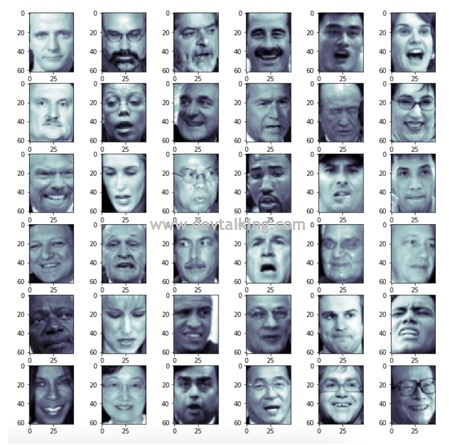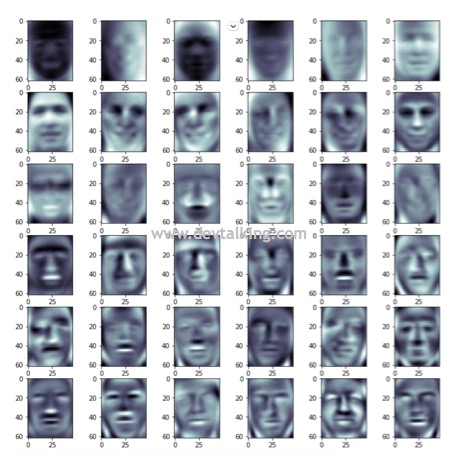• 机器学习算法收敛速度下降。
• 特征难于分辨，很难第一时间认识某个特征代表的意义。
• 会产生冗余特征，增加模型训练难度，比如说某一品牌型号汽车的特征数据，有从中国采集的，也有从国外采集的，那么就会产生公里/小时和英里/小时这种特征，但其实这两个特征代表的意义是一样的。
• 无法通过可视化对训练数据进行综合分析。

PCA是英文Principle Component Analysis的缩写，既主成分分析法。该算法能从冗余特征中提取主要成分，在不太损失模型质量的情况下，提升了模型训练速度。

理解PCA算法降维的原理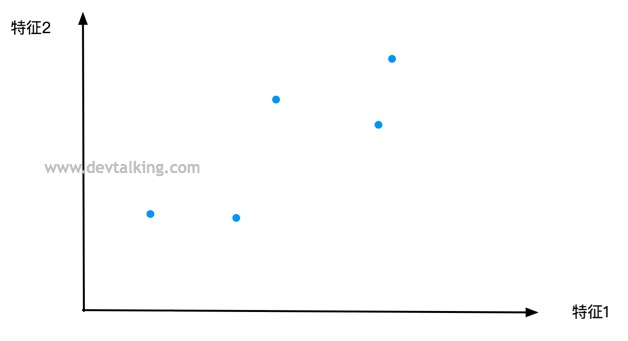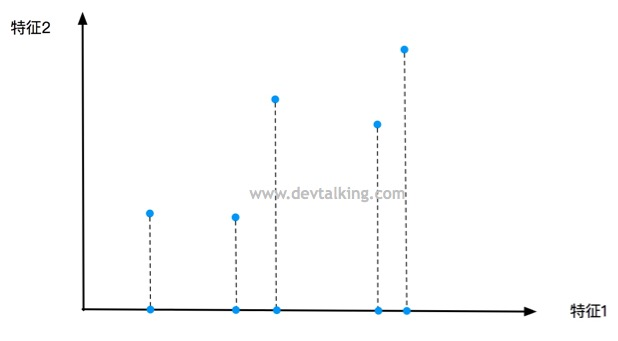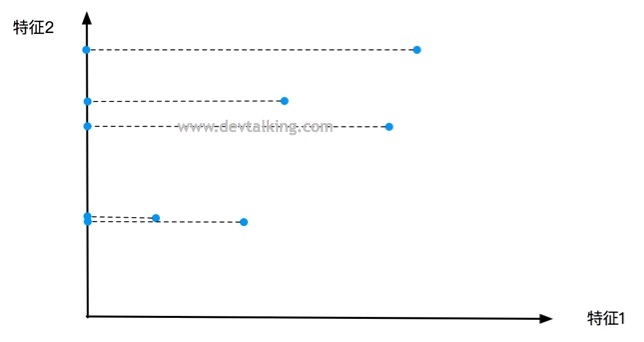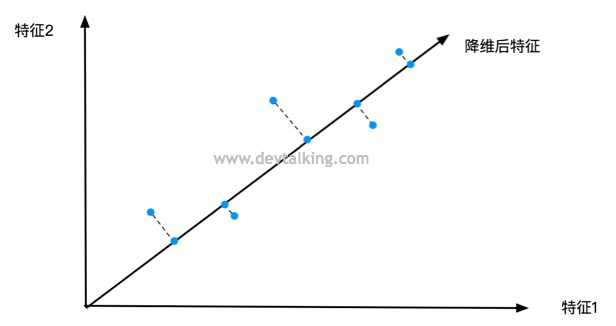$$Var(X) = \frac{\sum_{i=1}^m(X_i-\overline X)^2} m$$

$$Var(X) = \frac{\sum_{i=1}^mX_i^2} m$$

$$Var(X_p) = \frac{\sum_{i=1}^m (X_p^{(i)})^2} m$$

$$Var(X_p) = \frac{\sum_{i=1}^m ||X_p^{(i)}||^2} m$$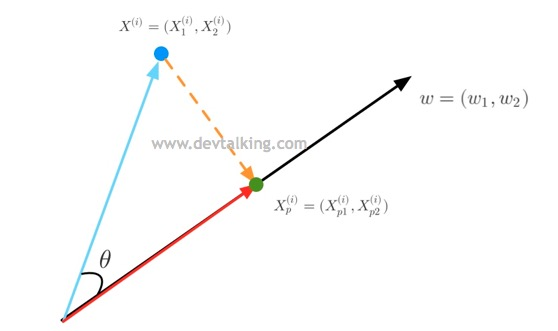$$\vec{a} \cdot \vec{b} = |\vec{a}| \, |\vec{b}| \cos \theta$$

$$X^{(i)} \cdot w=||X^{(i)}|| \cdot ||w|| \cdot \cos \theta$$

$$X^{(i)} \cdot w=||X^{(i)}||\cdot \cos \theta$$

$$||X_p^{(i)}||=||X^{(i)}|| \cdot \cos \theta$$

$$||X_p^{(i)}||=X^{(i)} \cdot w$$

$$Var(X_p) = \frac{\sum_{i=1}^m ||X^{(i)} \cdot w||^2} m$$

$$Var(X_p) = \frac{\sum_{i=1}^m (X^{(i)} \cdot w)^2} m$$

使用梯度上升法解决主成分分析问题

$$Var(X_p) = \frac{\sum_{i=1}^m (X^{(i)} \cdot w)^2} m\ =\frac 1 m \sum_{i=1}^m(X_1^{(i)}w_1+X_2^{(i)}w_2+…+X_n^{(i)}w_n)^2$$

$$\nabla f(w) = \begin{bmatrix} \frac {\partial L}{\partial w_1} \\ \frac {\partial L}{\partial w_2} \\ … \\ \frac {\partial L}{\partial w_n} \\ \end{bmatrix} =\frac 2 m\begin{bmatrix} \sum_{i=1}^m 2(X^{(i)}w)X_1^{(i)} \\ \sum_{i=1}^m 2(X^{(i)}w)X_2^{(i)} \\ … \\ \sum_{i=1}^m 2(X^{(i)}w)X_n^{(i)} \\ \end{bmatrix}$$

$$\begin{bmatrix}X^{(1)}w& X^{(2)}w& X^{(3)}w& … &X^{(m)}w)\end{bmatrix}$$

$$\begin{bmatrix}X^{(1)}w& X^{(2)}w& X^{(3)}w& … &X^{(m)}w)\end{bmatrix} \\ \cdot \begin{bmatrix} X_1^{(1)}& X_2^{(1)}& X_3^{(1)}& … &X_n^{(1)} \\ X_1^{(2)}& X_2^{(2)}& X_3^{(2)}& … &X_n^{(2)} \\ X_1^{(3)}& X_2^{(3)}& X_3^{(3)}& … &X_n^{(3)} \\ … \\ X_1^{(m)}& X_2^{(m)}& X_3^{(m)}& … &X_n^{(m)} \\ \end{bmatrix}$$

$$(Xw)^TX=\begin{bmatrix}\sum_{i=1}^m(X^{(i)}w)X_1^{(i)}& \sum_{i=1}^m(X^{(i)}w)X_2^{(i)}& …& \sum_{i=1}^m(X^{(i)}w)X_n^{(i)} \end{bmatrix}$$

$$((Xw)^TX)^T=X^T(Xw)$$

$$\nabla f = \frac 2 m X^T(Xw)$$

代码实现PCA梯度上升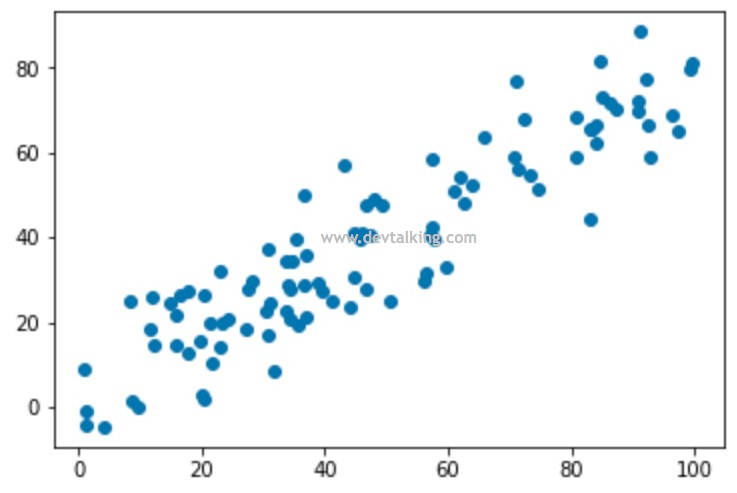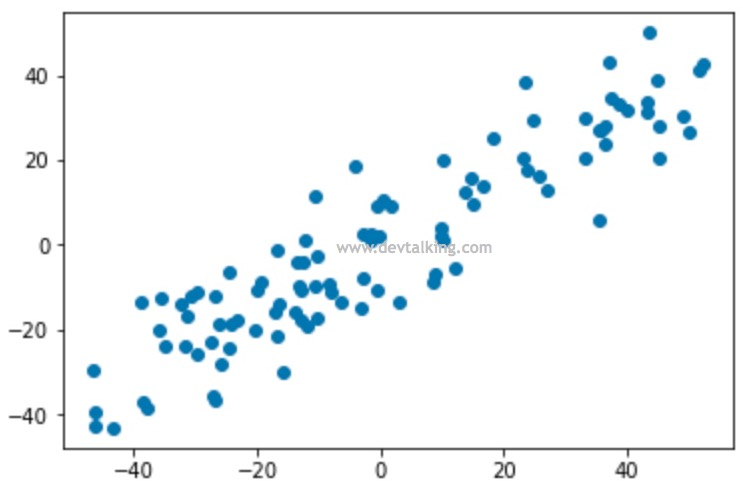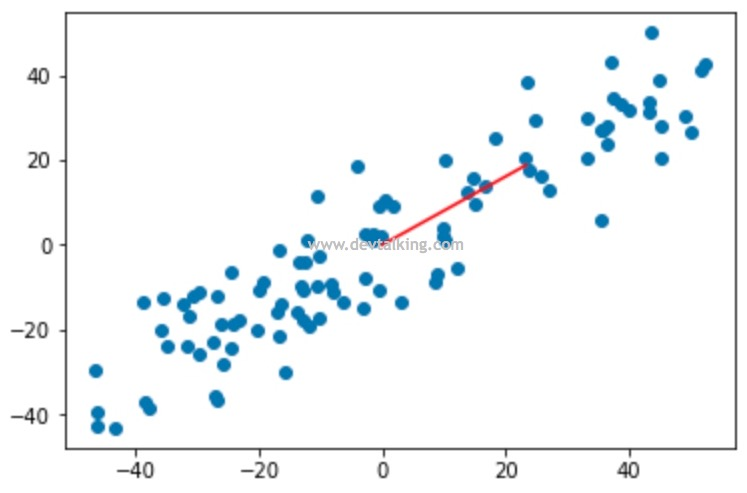求数据的其他主成分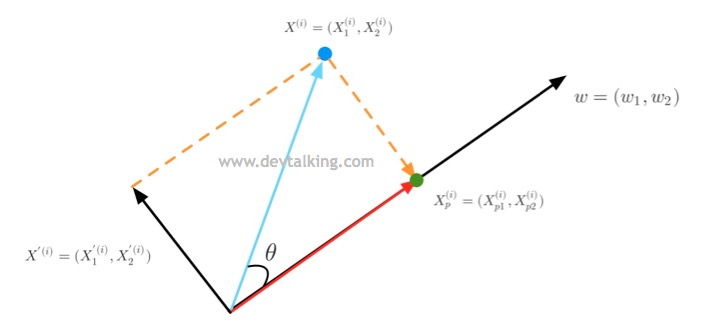$$X^{‘(i)}=X^{(i)}-X_p^{(i)}$$

$$X_p^{(i)}=||X_p^{(i)}|| w$$

$$||X_p^{(i)}||=X^{(i)} \cdot w$$

$$X^{‘(i)}=X^{(i)}-(X^{(i)} \cdot w)w$$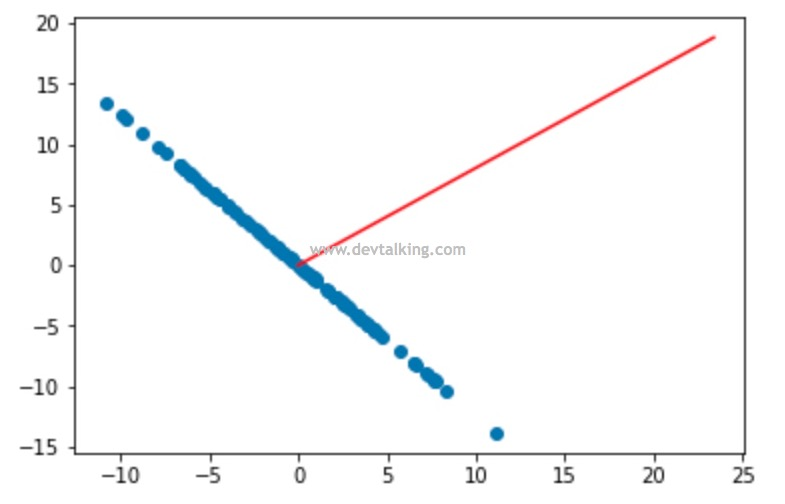高维数据向低维数据映射

$$X=\begin{bmatrix} X_1^{(1)}& X_2^{(1)}& … &X_n^{(1)} \\ X_1^{(2)}& X_2^{(2)}& … &X_n^{(2)} \\ … \\ X_1^{(m)}& X_2^{(m)}& … &X_n^{(m)} \end{bmatrix}$$

$$W_k=\begin{bmatrix} W_1^{(1)}& W_2^{(1)}& … &W_n^{(1)} \\ W_1^{(2)}& W_2^{(2)}& … &W_n^{(2)} \\ … \\ W_1^{(k)}& W_2^{(k)}& … &W_n^{(k)} \\ \end{bmatrix}$$

$$||X_p^{(i)}||=X^{(i)} w$$

$$X^{‘}=XW_k^T$$

$X^{‘}$就是降维后的数据，既然可以降维，那么我们也可从数学的角度将降维后的数据还原回去。$X^{‘}$是m行k列的矩阵，$W_k$是k行n列的矩阵，所以$X^{‘} W_k$就是还原后的ｍ行ｎ列的原矩阵。那为什么说是从数学角度来说呢，因为毕竟已经从高维降到了低维，那势必会有丢失的数据信息，所以还原回去的数据也不可能和原始数据一样的。

在Jupyter Notebook中使用封装的PCA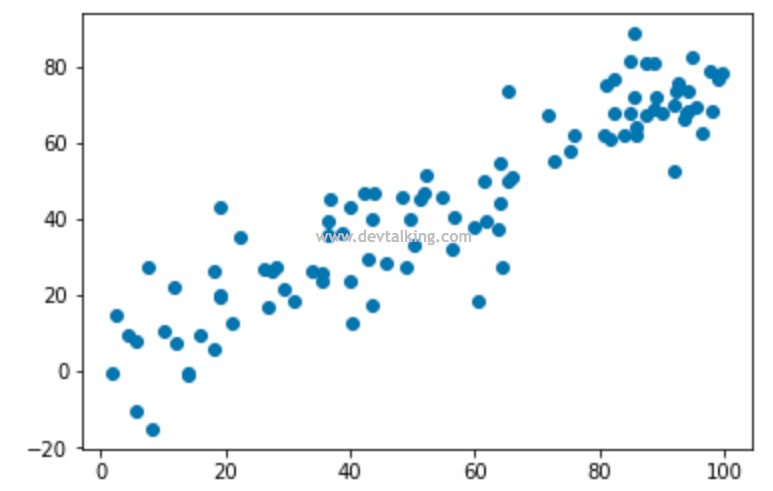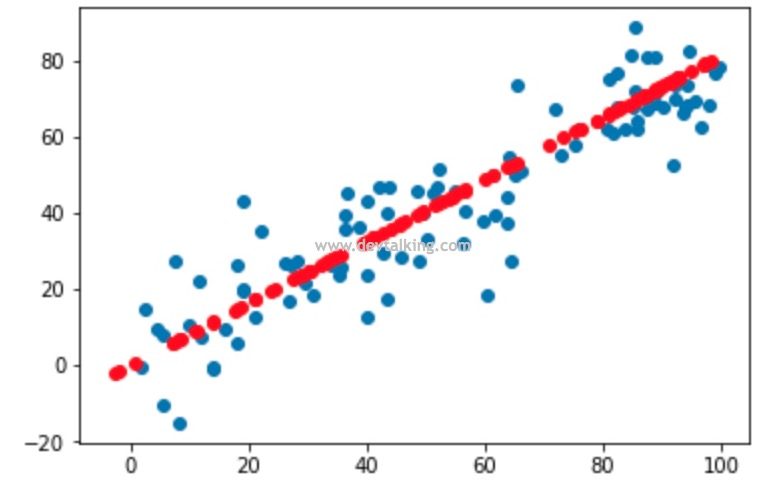Scikit Learn中的PCA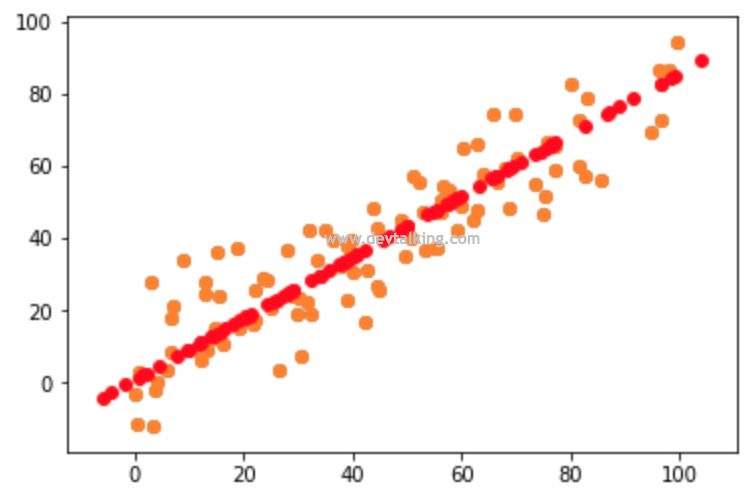使用真实的数据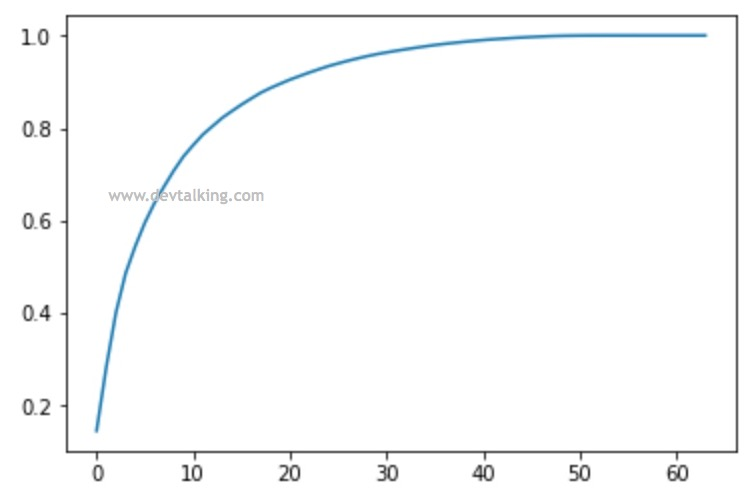使用PCA对数据进行降噪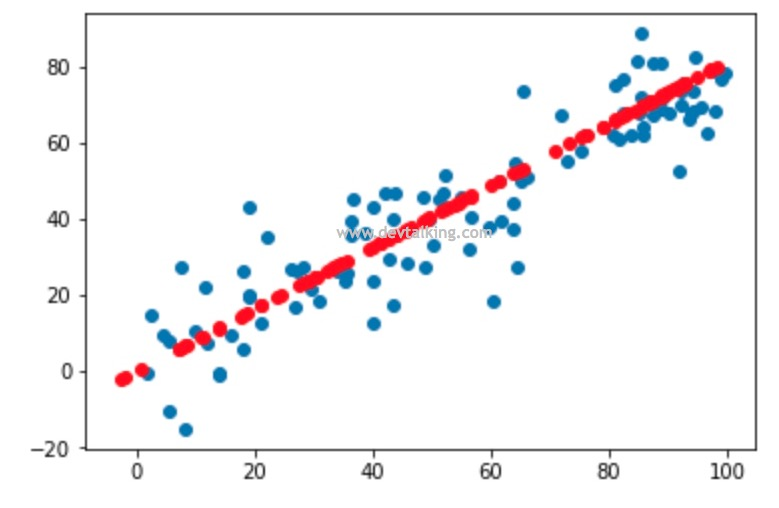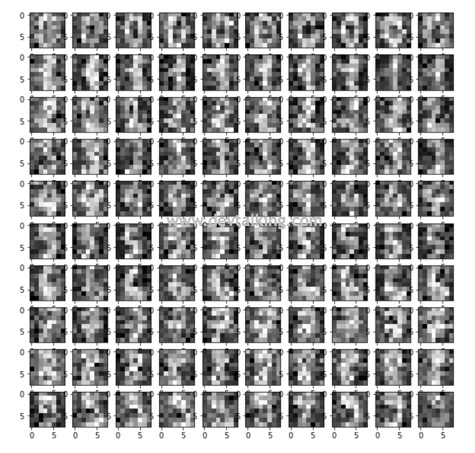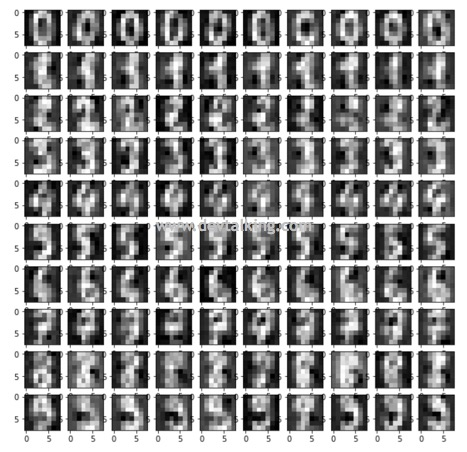人脸识别

PCA有一个典型的实际应用，就是人脸识别。我们这一节就来简单看看PCA在人脸识别中的应用。